# Practice Problems with Circular Trigonometric Functions

Lesson Transcript
Instructor: Yuanxin (Amy) Yang Alcocer

Amy has a master's degree in secondary education and has taught math at a public charter high school.

After watching this video lesson, you will be able to use the unit circle to help you find your answer to trig problems without making too many calculations. Watch and learn how you can find your angle in radians on the unit circle.

## The Unit Circle

In this video lesson, we are dealing with circular trigonometric functions. These are your trig functions calculated using a circle. What kind of circle? We will use a special circle called a unit circle. We call this special circle a unit circle because it has a radius of 1. If we plot this on our Cartesian coordinate graph, the center of our circle will be at the origin. The circle crosses the x axis on the right side at (1, 0) and on the left side at (-1, 0). The circle also crosses the y axis on the top at (0, 1) and on the bottom at (0, -1).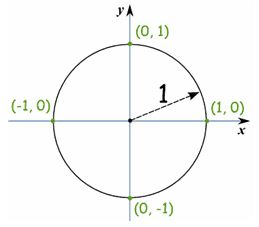Using the unit circle to help solve our trig functions means that all of our points will be somewhere on the unit circle. Where do the angles come into play with the unit circle? This is a good question. If we take a point, we can draw a line from the center of our circle to the point. We will have a radius of 1. The angle formed by this line and our positive x axis is the angle that we use for our calculations. The real neat part about the unit circle is that our x value then becomes cos (theta) and our y value is sin (theta).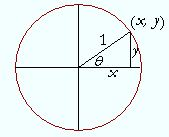How does this work? Remember our definitions of our cosine and sine functions using a right triangle? Cosine is adjacent over hypotenuse. Looking at our unit circle and the triangle that is formed by the radius, the x value and the y value of our point, we see that our adjacent is x and our hypotenuse is 1. So adjacent over hypotenuse is then x/1 or x. We have cos (theta) = x/1 = x.

As for the sine function, we have sin (theta) equals opposite over hypotenuse. Our opposite is y and our hypotenuse is 1. So sin (theta) = y/1 = y. Pretty neat, huh? This helps us out a lot because we can actually fill in our unit circle with various points that are easy to calculate.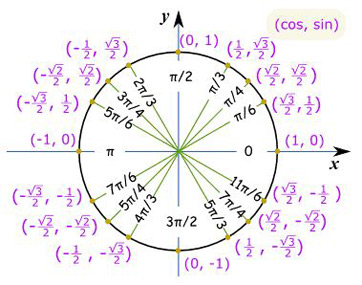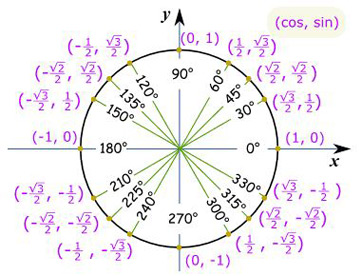Just look at all these points! If you can remember these, then you are well on your way to becoming a circular trig function pro! How can you remember all of these points? They look a bit complicated, don't they? They do, but if you see the pattern that is there, then you won't have as much trouble.

Look carefully at the numerators. Now look at what the points are doing. As you go from point to point, you see that your numbers are actually increasing or decreasing by 1. You still have your square root, but the number inside is going from 1 to 2 to 3 and back again. It is the same for both the x and y values.

As you go higher up on the y axis, the numerator for the y value goes from 1 to the square root of 2 to the square root of 3. To help you with this, you can also think of the 1 as the square root of 1 so that you have all square roots. You can see that the numbers follow the coordinate graph.

As the x value goes more negative, so does the numerator of the x value. It goes from -1 to the square root of -2 to the square root of -3. As for the denominator, all the points that aren't the easy points like (0, 1) have a denominator of 2. That's all you have to remember.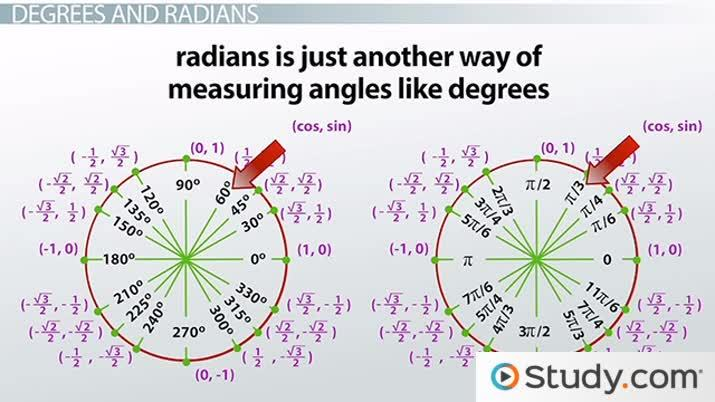An error occurred trying to load this video.

Try refreshing the page, or contact customer support.

Coming up next: Special Right Triangles: Types and Properties

### You're on a roll. Keep up the good work!

Replay
Your next lesson will play in 10 seconds
• 0:01 The Unit Circle
• 4:13 Example 1
• 5:03 Example 2
• 5:36 Example 3
• 6:57 Lesson Summary
Save Save

Want to watch this again later?

Timeline
Autoplay
Autoplay
Speed Speed

Okay, that's all well and good, but what's with the two different graphs? One has a whole lot of pis in it. Another good question! This second graph with the pis in it is showing you the equivalent radian measure of the degrees. So we have 90 degrees is the same as pi/2 radians and 60 degrees is pi/3 radians.

See, radians is just another way of measuring angles like degrees. You will see on your calculator that you can choose between the two when making calculations. Make sure you are using the correct one. Use radians when working with radians and use degrees when working with degrees. Otherwise, you'll get a whole bunch of bologna or nonsense for your answers. I've shown you both of these graphs because many times you will see circular trigonometric functions used with radians instead of degrees. If you know the radian measure, then you can easily find your answer. Many times, you will be asked to find one of the angles that are specifically marked on the unit circle. So knowing your radian measure along with the unit circle will give you that much of a head start in solving problems.

## Example 1

Let's take a look at what you might expect to find on tests and such. You might come across a problem that asks you something like this:

To unlock this lesson you must be a Study.com Member.

### Register to view this lesson

Are you a student or a teacher?